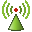# Requesting Specific Absorption Rate (SAR)

Add a request to calculate the average absorption over a volume (volume-average SAR) or the maximum absorption in a 1 g or 10 g cube in a given volume (spatial-peak SAR).

1. On the Request tab, in the Solution Requests group, click theSAR icon.
2. Under Select calculation, select the type of SAR calculation:
• To calculate the average absorption over a volume, select Volume-average SAR.
• To calculate the maximum absorption in a 1g cube in the model, select Spatial-peak SAR of a 1g cube.
• To calculate the maximum absorption in a 10g cube in the model, select Spatial-peak SAR of a 10g cube.
3. Specify the region where the SAR is calculated. Under Specify the search region, select one of the following:
• To calculate SAR in all the dielectric regions in the model and calculate a single average or peak SAR value, select Entire model.
• To calculate SAR in all media or a specified medium, select By medium.
• To calculate SAR in a specific layer or in all the layers of a planar substrate, select In a planar substrate.
Note:
• Layer 0 is the upper free space region.
• Layer 1 is the uppermost dielectric layer.
• To calculate the 1g or 10g cube SAR at a specified location, select At a specified position. This option is not available for volume average SAR.
4. In the Label field, add a unique label for the request.
5. Click Create to request the SAR result and to close the dialog.